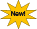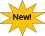site search by freefind

## Statistical data analysis - Christoph Scherber

• Workshops &
Courses
• Introduction to Statistics
and R
• R scripts
& Code
Channel

July 2019

Course on mixed effects models will be given at the GfÖ conference in Münster

October 2017

MSc-level statistics course in English language (Umweltstatistik II - Analysis of scientific experiments)

October 2014

PhD course "Linear statistical models with R", Göttingen; Room L318, Grisebachstrasse 6, 6th-10th October

June 2014

Workshop with John Fox on structural equation modelling on 6th June 2014. John Fox is an eminent expert in linear statistical models and he´s written books such as An R Companion to Applied Regression.

April 2014

Workshop by Christoph Scherber "Structural equation models in the Soil Sciences", Deutsche Bodenkundliche Gesellschaft

February 2014

Workshop "Structural Equation models" organized by C. Scherber. Guest speakers and lecturers:

James B. Grace (USGS)
W. Daniel Kissling (Denmark & The Netherlands)
Niels J. Blunch (Denmark)

Statistical graphicsUsing R in combination with Adobe Illustrator CS6 for professional graphics outputpublished in Software Developer´s Magazine 4/2012.

General introductions to R and to statistical data analysis

An Introduction to Statistical Data analysis

An Introduction to R

Linear models

Non-linear mixed-effects models in R

Linear models in matrix notation

Log-transformation for data assuming negative values:

log.modulus=function(x,k)sign(x)*log(abs(x)+k)
# k is an arbitrary constant to avoid taking logs of zero

Christoph´s mixed R functions

This is a continuously updated file containing useful snippets of R code.

Multinomial models

When fitting multinomial models with the nnet package (multinom() function), it is sometimes desirable to increase the number of weights (especially when there is a large number of response categories). This may happen for example in the analysis of next-generation sequencing data. The Anova() function from John Fox´s package "car" can not deal with the MaxNWts argument and hence cannot be used for multinomial models with user-specified maximum number of weights. Below, I provide a function called Anova.multinom2, which allows MaxNWts to be set to any desirded number.Anova for models with user-specified weights, fitted with the multinom() function

Nonlinear regression

In nonlinear regression situations, one often wishes to use power law functions of the form y=a+b*x^c. The following code allows starting estimates for this function to be estimated automatically.Self-starting non-linear power law function in R

Model selection

StepAICc function for linear, generalized linear and mixed models

selMod function for model selection in mixed models

Contrast matrices

Working with orthogonal contrasts in R

Test for orthogonality of a contrast matrix

Linear mixed models

Extract lme ANOVAs from multiple models

Extract lme summaries from multiple models

Generalized linear mixed models

ANOVA method for lmer models

Time series analysis

A short introduction to time-series analysis in R

Graphics

Creating publication-quality R Graphics

Ecological diversity

Calculate Shannon´s diversity index

Seed bank data analysis

Analyzing data from a seed bank study using R

Watch videos from my new YouTube channel:

Please contact me if you feel there are things that would need to be corrected. R is open-source and new libraries are published every other day, and so it is always a challenging task to keep up with all new developments.

Thanks to the R Core Development Team for making R possible, and also to Mick Crawley for introducing me to R. For downloading and installing R, please visit the R Project website.